A wire of resistance R is cut into ‘n’ equal parts. These parts are then connected in parallel. The equivalent resistance of the combination will be :

(1) nR

(2) $\frac{R}{n}$

(3) $\frac{n}{R}$

(4) $\frac{R}{{n}^{2}}$

Concept Questions :-

Combination of resistors
High Yielding Test Series + Question Bank - NEET 2020

Difficulty Level:

The resistance between the terminal points A and B of the given infinitely long circuit will be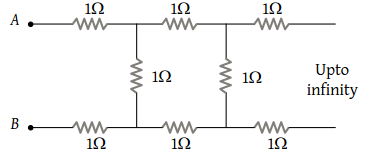(1) $\left(\sqrt{3}-1\right)$

(2) $\left(1-\sqrt{3}\right)$

(3) $\left(1+\sqrt{3}\right)$

(4) $\left(2+\sqrt{3}\right)$

Concept Questions :-

Combination of resistors
High Yielding Test Series + Question Bank - NEET 2020

Difficulty Level:

The current in the given circuit is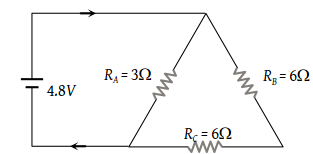(1) 8.31 A

(2) 6.82 A

(3) 4.92 A

(4) 2 A

Concept Questions :-

Combination of resistors
High Yielding Test Series + Question Bank - NEET 2020

Difficulty Level:

What is the current (i) in the circuit as shown in figure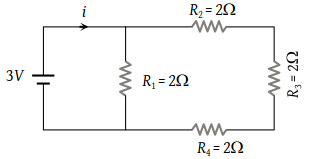(1) 2 A

(2) 1.2 A

(3) 1 A

(4) 0.5 A

Concept Questions :-

Combination of resistors
High Yielding Test Series + Question Bank - NEET 2020

Difficulty Level:

n equal resistors are first connected in series and then connected in parallel. What is the ratio of the maximum to the minimum resistance

(1) n

(2) $\frac{1}{{n}^{2}}$

(3) n2

(4) $\frac{1}{n}$

Concept Questions :-

Combination of resistors
High Yielding Test Series + Question Bank - NEET 2020

Difficulty Level:

In the figure, current through the 3 Ω resistor is 0.8 ampere, then potential drop through 4 Ω resistor is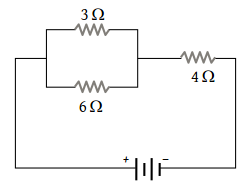(1) 9.6 V

(2) 2.6 V

(3) 4.8 V

(4) 1.2 V

Concept Questions :-

Combination of resistors
High Yielding Test Series + Question Bank - NEET 2020

Difficulty Level:

What will be the equivalent resistance between the two points A and D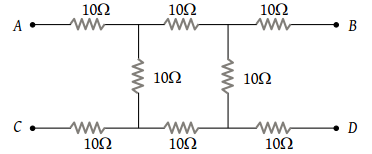(1) 10 Ω

(2) 20 Ω

(3) 30 Ω

(4) 40 Ω

Concept Questions :-

Combination of resistors
High Yielding Test Series + Question Bank - NEET 2020

Difficulty Level:

What is the equivalent resistance between A and B in the figure below if R = 3 Ω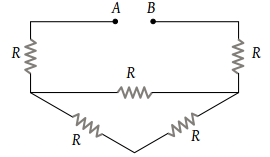(1) 9 Ω

(2) 12 Ω

(3) 15 Ω

(4) None of these

Concept Questions :-

Combination of resistors
High Yielding Test Series + Question Bank - NEET 2020

Difficulty Level:

What is the equivalent resistance between A and B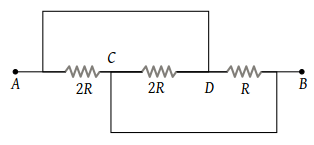(1) $\frac{2}{3}R$

(2) $\frac{3}{2}R$

(3) $\frac{R}{2}$

(4) 2 R

Concept Questions :-

Combination of resistors
High Yielding Test Series + Question Bank - NEET 2020

Difficulty Level:

The current in the following circuit is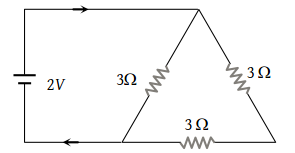(1) $\frac{1}{8}A$

(2) $\frac{2}{9}A$

(3) $\frac{2}{3}A$

(4) 1A

Concept Questions :-

Combination of resistors